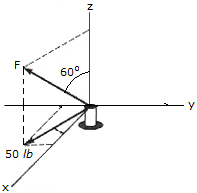# Engineering Mechanics - Force Vectors - Discussion

### Discussion :: Force Vectors - General Questions (Q.No.5)

5.Force F acts on peg A such that one of its components, lying in the x-y plane, has a magnitude of 50 lb. Express F as a Cartesian vector.

 [A]. F = (43.3 i + 25.0 j + 25.0 k) lb [B]. F = (43.3 i + 25.0 j + 28.9 k) lb [C]. F = (43.3 i - 25.0 j + 25.0 k) lb [D]. F = (43.3 i - 25.0 j + 28.9 k) lb

Explanation:

No answer description available for this question.

 Aimen said: (Nov 28, 2014) Please give me the solution.

 Riyaz Ibrahim said: (Oct 8, 2015) Go through F = 50sin60-50cos60+50tan60.

 Ameet Kumar said: (Feb 16, 2016) Kindly sir please give me the specific solution?

 Gershom Ntsako said: (Mar 18, 2016) 50 = Fcos30. F = 57.74. 57.74sin(30) = Z-COMPONENT. = 28.867. THEREFORE x-component should be +ve and y-component will be -ve.

 Manoj said: (Feb 28, 2017) We know that x component of the force F is F(x) = Fcos30. From that F = F(x)/cos30= 57.77 From the figure, we come to know that the direction of the force is in +ve x direction -ve y direction and +ve z direction. Then take the two options which have negative j. Ffind z component F(y)k= Fcos60 z = 57.7*cos60=28.86k. Hence Option D is correct.

 Anonymous said: (Nov 3, 2019) How come everyone see 30 degree? I only see 60 degree only.

 Anonymous said: (Feb 13, 2020) See, F is making 60 degrees with the z-axis, but the question says "one of its components lying in the XY axis has a magnitude of 50". Therefore, we consider F making 30 degrees with X-axis (90-60 = 30) which gives => 50 = FCos30.